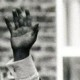# A puzzle from Donald KnuthRecently I had to write some code to generate a pseudorandom number in a system with very limited sources of entropy. So, of course I turned to Donald Knuth and, in particular, Volume 2 – Seminumerical Algorithms – in the magisterial The Art of Computer Programming.

Reading through the questions/exercises I then came across this one:

Prove that the middle-square method using 2n-digit numbers to the base b has the following disadvantage: if the sequence includes any number whose most significant n digits are zero, the succeeding numbers will get smaller and smaller until zero occurs repeatedly.

(Knuth rates this question as ’14’ and, using his scale of difficulty which places a ’10’ as a minute to solve and a 20 as twenty minutes, probably means this should take about 7 or 8 minutes but I’ve spent much, much longer on it than that!)

A quick explanation: the middle-square method is a naive (though actually first suggested by none other than John von Neumann) random number generation method where we take a number n-digits long, square it and take the middle n-digits as our next seed or random number.

At first I thought I’d found an example where Knuth’s proposition appears to be false.

Let$b=10$ and the seed number be$N_0 =60$ then every subsequent number in the sequence is also 60 (obviously that’s as useless as repeated zeros for a random number generator.) But the problem with that is, that although it demonstrates a weakness in the middle square method, it doesn’t fit Knuth’s definition of the problem. What is$n$ here? If$n = 2, 2n=4, 4n=8$ ($n=2$ is the minimum for middle values), then $N_o = 0060$ and$N_0^2 = 00003600$ and so$N_1 = 0036, N_2=0012, N_3=0001, N_4=0$ (thanks to Hagen von Eitzen for clarifying this for me.)

So let’s look at the general case. (I also this explanation to Hagen von Eitzen, as compared to my very long-winded first attempt – though any errors that follow are mine not his.)

So we have a number$x$ which we think of as a$2n$ digit number – though the first$n$ digits are 0. Then$x^2 < b^{2n}$ (as the largest$x$ can be is$b^n -1$.)

Thus as the largest$x$ can be is$b^n - 1$ then:$\frac{x^2}{b^n} \leqslant \frac{x(b^n - 1)}{b^n}$

And$\frac{x(b^n - 1)}{b^n} = x-\frac{x}{b^n}$

If we have a$2n$ digit number$x$ then the biggest number of digits we can get from the out put is$4n$, but in our case we only have$n$ digits to worry about so the biggest size$x^2$ can be is$2n$ digits, as again the leading$2n$ digits will be 0.

So, to apply the middle square method we need to lose the lower$n$ digits – i.e., take$\lfloor\frac{x^2}{b^n}\rfloor$.

From the above:$\lfloor\frac{x^2}{b^n}\rfloor \leqslant \lfloor x-\frac{x}{b^n} \rfloor$

If$x > 0$ then$\lfloor x-\frac{x}{b^n}\rfloor$ will always$< x$, so the sequence decreases until$x = 0$.Talk to the hand